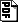## Minimum Circuit Size, Graph Isomorphism, and Related Problems#### Abstract

We study the computational power of deciding whether a given truth-table can be described by a circuit of a given size (the Minimum Circuit Size Problem, or MCSP for short), and of the variant denoted MKTP where circuit size is replaced by a polynomially-related Kolmogorov measure. All prior reductions from supposedly-intractable problems to MCSP / MKTP hinged on the power of MCSP / MKTP to distinguish random distributions from distributions produced by hardness-based pseudorandom generator constructions. We develop a fundamentally different approach inspired by the well-known interactive proof system for the complement of Graph Isomorphism (GI). It yields a randomized reduction with zero-sided error from GI to MKTP. We generalize the result and show that GI can be replaced by any isomorphism problem for which the underlying group satisfies some elementary properties. Instantiations include Linear Code Equivalence, Permutation Group Conjugacy, and Matrix Subspace Conjugacy. Along the way we develop encodings of isomorphism classes that are efficiently decodable and achieve compression that is at or near the information-theoretic optimum; those encodings may be of independent interest.

dieter@cs.wisc.edu